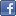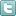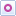EE Times-India > T&M

T&M# How to measure frequency response on a scope

Posted: 22 Dec 2015Print Version

Keywords:Oscilloscopes  Fourier Transform  FFT  Swept sine waves  arbitrary waveform generator

Oscilloscopes are time-domain instruments, however they can process time-domain signals into the frequency domain because they digitise waveforms. The Fast Fourier Transform (FFT), spectrum analyser options, and similar frequency domain tools, let you measure a circuit's frequency response with an oscilloscope. You can use several types of input excitation signals to measure frequency response: step functions, swept sines waves, white noise, and phase-modulated carriers.

The simplest type of frequency response measurement requires a signal source with a spectrally "flat" response in the frequency domain. This means that the signal spectrum is constant across all frequencies of interest. Swept sine waves, white random noise, and the Dirac impulse function all provide flat responses and all are available in arbitrary waveform generators. Keeping in mind that the impulse function is the derivative of a step function, it is also possible to use a fast edge or step signal from the oscilloscope to do frequency response testing. This later fact means that the oscilloscope can make self-contained frequency response measurements.

Step function
Our first example uses the step function to excite a digital filter, from which we measure frequency response. Figure 1 shows all the steps involved in this measurement.Figure 1: An FFT function lets you measure the frequency response of a digital filter that's excited by a step-signal source.

In figure 1, we use a step waveform as the input signal. The oscilloscopes "fast edge" or "cal" signal could be used depending on the desired measurement bandwidth. The signal's bandwidth is inversely proportional to the step's transition time. To make the measurement, apply the input signal to channel 1, shown in the upper left trace.

Math trace F4, just below the trace of the input signal, differentiates the step input. This is an impulse function. Note that the derivative math function is sensitive to baseline noise, which you can counter by decreasing the effective sample rate using the oscilloscope's sparse function. You can reduce the sample rate to a minimum of twice the desired measurement bandwidth and still satisfy the Nyquist Criteria.

The next lower trace is the FFT of the impulse. It shows a flat frequency spectrum over the 100MHz band of interest. Cursor measurements show that the spectrum of the impulse function is flat to within 20 mdB over the bandwidth of the filter being tested. The step function is also applied to the input of the digital filter. The filter's output is shown in trace F1, in the upper right. This is differentiated in trace F2 (right centre). The differentiated output is applied to the averaged FFT in trace F3 (right bottom), which shows the output signal's spectrum.

Technically, the frequency response is the complex ratio of the output spectrum to the input spectrum. Since we have assured that the input spectrum magnitude is flat, the output spectrum represents the correct shape of the frequency response. Cursors measure the -3 dB point as having a frequency of 38MHz.

Swept sine
The example shown in figure 2 uses a swept-sine input from an arbitrary waveform generator. The sweep is set up to cover 10kHz to 200MHz.Figure 2: Using a swept sine to measure the frequency response of a digital filter results in a small "notch" as the input excitation signal starts (see centre-right trace).

 Related Articles Editor's Choice
Comment on "How to measure frequency response on..."

Top Ranked Articles

WebinarsVisit Asia Webinars to learn about the latest in technology and get practical design tips.

Search EE Times India
Services

Go to topConnect on FacebookFollow us on TwitterFollow us on Orkut
﻿